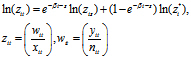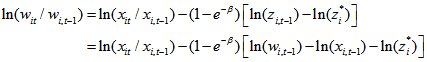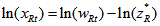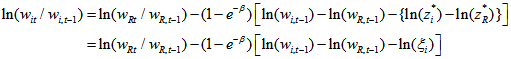# 2. Theory

Date

ï»¿

## The basic neo-classical growth model

The basic neo-classical model (Ramsey model) provides the basis for much of the empirical growth literature. This is due to its parsimony and broad consistency with observed data. In particular, Barro and Sala-i-Martin (1992) show that the near steady-state dynamics of output per unit of effective labour of country i at time t can be approximated by the following dynamic relationship:where yit is output in country i at time t, nit is country i labour input at time t, wit is country i labour productivity at time t, xit country i labour augmenting technology at time t, zit is country i output per effective labour at time t, with an * indicating steady-state values and β is the speed of convergence. The speed of convergence depends on long-run technology and preference parameters which we assume are common to all countries.

This implies that the per-period growth rate of labour productivity is governed by the following error correction framework:According to this relationship, productivity growth is a function of growth of labour-augmenting technology and the percentage deviation of actual output per effective labour unit from its steady-state level. Along the balanced growth path, output per effective unit of labour is equal to its steady-state value so labour productivity will grow at the same rate as labour-augmenting technology. If a country is below its steady-state level of output per effective labour unit then its productivity will grow at a faster rate than labour-augmenting technology.

A common working and empirical assumption is that countries have the same rate of growth of exogenous technological change, which implies they have the same steady-state growth rate of per capita income. Heterogeneity is introduced by assuming that countries have the same level of labour-augmenting technology but potentially different steady-state output per unit of effective labour (that is, different steady-state ratios of labour productivity to common labour augmenting technology). Without loss of generality we can assume that there is a reference country (denoted by i=R) that is growing along its balanced growth path (that is, the reference country’s labour productivity grows at the same rate as labour augmenting technology):Substituting (3) into (2) yields the following relationship between the reference and other country’s productivity:where ξi=zi*/zR* is the relative productivity of country i.

It follows that the productivity growth rate of a country i will be higher than the productivity growth rate of the reference country when country i’s actual relative productivity is below its steady-state value, which is equal to the reference country’s productivity scaled by country i’s relative steady-state productivity level (that is, ξiwR).

Country i converges absolutely to the reference country if ξi is one and converges conditionally to the reference country if ξI is less than or greater than one. Empirical studies typically use the United States (US) as the reference country because US productivity has grown persistently over the past 100 years and it tends to be higher than that of other advanced countries. Following this approach, a country’s level of conditional convergence is measured as a proportion of US productivity (that is, X per cent of US productivity, hereafter referred to as the steady-state relative productivity). The empirical growth literature has identified a number of factors that are correlated with the measures of conditional convergence which are surveyed by Barro and Sala-i-Martin (2004).